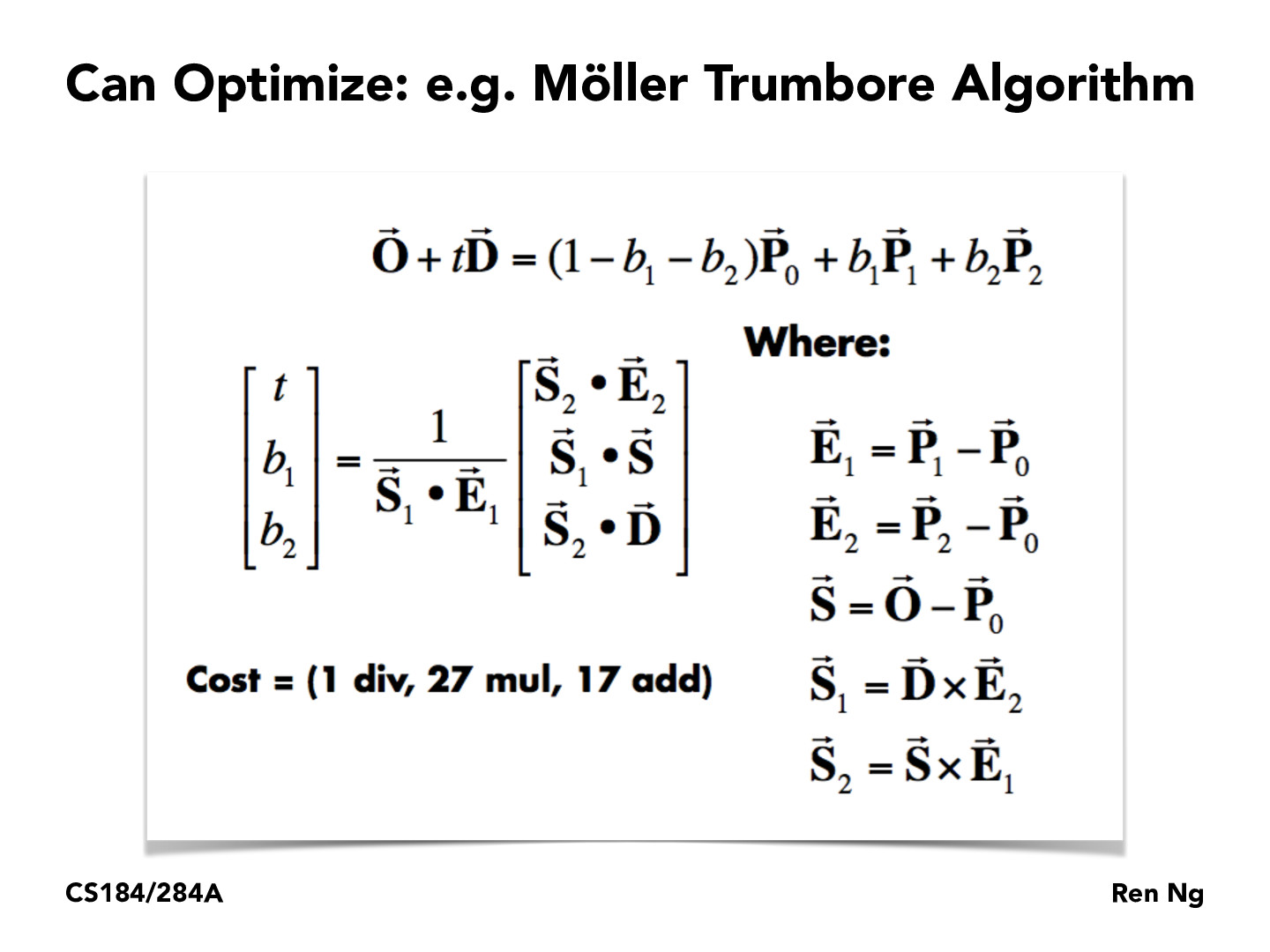You are viewing the course site for a past offering of this course. The current offering may be found here.Lecture 10: Ray Tracing (20)FLinesse

For those interested: https://www.scratchapixel.com/lessons/3d-basic-rendering/ray-tracing-rendering-a-triangle/moller-trumbore-ray-triangle-intersectiongavinmak

How is the cost compared across divisions, multiplications, and additions? i.e. is there a single value that could be calculated to determine how fast it would be without actually running the algorithm? Would that depend on the computer used to calculate the algorithm?ABSchloss

Different operations are sometimes different speeds, depending on the implementation for that language. You can determine asymptotic speeds based of input sizes if you look at different algorithms used. Such as https://en.wikipedia.org/wiki/Karatsuba_algorithm, which speeds up multiplication from n^2 to n^(log_2(3)).frgalvan

so if 0 <= b1 <= 1 && 0 <= b2 <= 1 && b1 + b2 <= 1, its safe to say the point lies inside the triangle? it This exhaustive? or does it require some sort of near by (epsilon) factor for accuracy?frgalvan